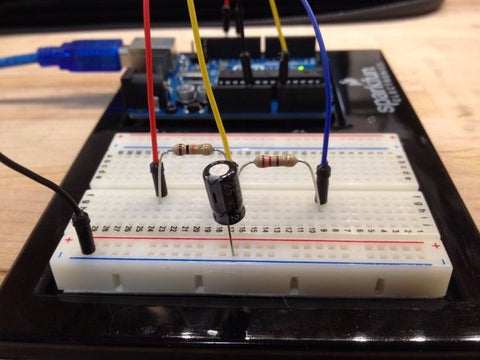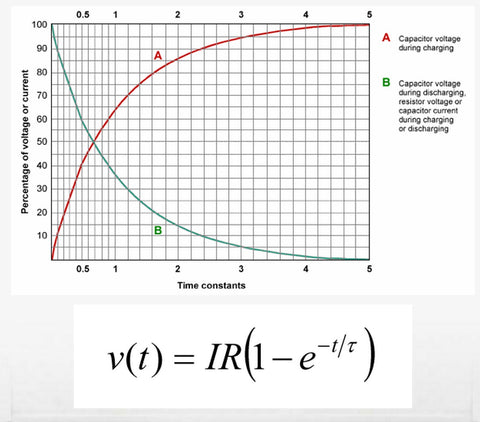# Tutorial: Measure Capacitance with An Arduino

July 14, 2015Max Kennard shared this Instructables tutorial for using an Arduino to measure the capacitance of a unlabeled or custom-built cap in your parts box:

...Capacitance is an object's ability to store an electric charge. Reasonably, this object is referred to as a capacitor. A capacitor that stores this charge in an electric field between two conductive plates is known as a parallel plate capacitor. The non-conductive material that is between these two plates is known as a dielectric. Dielectrics change the amount of charge a capacitor can hold and , in practice, what the particular capacitor would be used for (e.g. high frequency circuits, high voltage circuits, etc)....
The Arduino will be programmed to time how long it takes for a capacitor to reach 63.2% of its total charge. It will then use the equation for Tau to calculate the capacitance since the value of the resistor is already known....Author